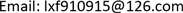MnCo<sub>2</sub>O<sub>4</sub>@Ni(OH)<sub>2</sub>复合材料的制备 及作为超级电容器正极材料的性能研究<sup> </sup>1. 引言

2. 实验2.1. MnCo<sub>2</sub>O<sub>4</sub>@Ni(OH)<sub>2</sub>复合材料的制备

1) MnCo2O4的制备

2) MnCo2O4@Ni(OH)2复合材料的制备

2.2. 材料表征

2.3. 电化学性能测试

C s = I × Δ t / ( Δ v × m 1 ) (1)

C s = I × Δ t / ( Δ v × m 2 ) (2)

3. 结果与讨论

MnCo 2 O 4 + H 2 O + oH − ⇌ MnOOH + 2 CoOOH + e − (3)

Ni(OH)2发生的反应表述如下：

Ni ( OH ) 2 + oH − ⇌ NiOOH + H 2 O + e − (4)

m − / m + = ( c + × Δ v + ) / ( c − × Δ v − ) (5)

E = C V 2 / 2 (6)

P = E / Δ t (7)

4. 结论